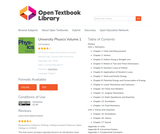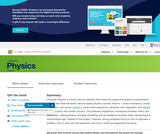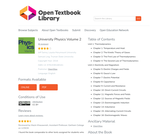# 4 Results

View
Selected filters:
• Samuel J. LingUnrestricted Use
CC BY
Rating

University Physics is a three-volume collection that meets the scope and sequence requirements for two- and three-semester calculus-based physics courses. Volume 1 covers mechanics, sound, oscillations, and waves. Volume 2 covers thermodynamics, electricity and magnetism, and Volume 3 covers optics and modern physics. This textbook emphasizes connections between between theory and application, making physics concepts interesting and accessible to students while maintaining the mathematical rigor inherent in the subject. Frequent, strong examples focus on how to approach a problem, how to work with the equations, and how to check and generalize the result.

Preface
Unit 1. Mechanics

Chapter 1: Units and Measurement
Chapter 2: Vectors
Chapter 3: Motion Along a Straight Line
Chapter 4: Motion in Two and Three Dimensions
Chapter 5: Newton's Laws of Motion
Chapter 6: Applications of Newton's Laws
Chapter 7: Work and Kinetic Energy
Chapter 8: Potential Energy and Conservation of Energy
Chapter 9: Linear Momentum and Collisions
Chapter 10: Fixed-Axis Rotation
Chapter 11: Angular Momentum
Chapter 12: Static Equilibrium and Elasticity
Chapter 13: Gravitation
Chapter 14: Fluid Mechanics
Unit 2. Waves and Acoustics

Chapter 15: Oscillations
Chapter 16: Waves
Chapter 17: Sound
Appendix A: Units
Appendix B: Conversion Factors
Appendix C: Fundamental Constants
Appendix D: Astronomical Data
Appendix E: Mathematical Formulas
Appendix F: Chemistry
Appendix G: The Greek Alphabet
Index

Subject:
Physics
Material Type:
Textbook
Provider:
Rice University
Provider Set:
OpenStax College
Author:
Alice Kolakowska
Bill Moebs
Daniel Bowman
David Anderson
David Smith
Dedra Demaree
Edward S. Ginsberg
Gerald Friedman
Joseph Trout
Kenneth Podolak
Kevin Wheelock
Lee LaRue
Lev Gasparov
Mark Lattery
Patrick Motl
Richard Ludlow
Samuel J. Ling
Takashi Sato
Tao Pang
08/03/2016Unrestricted Use
CC BY
Rating

University Physics is a three-volume collection that meets the scope and sequence requirements for two- and three-semester calculus-based physics courses. Volume 1 covers mechanics, sound, oscillations, and waves. Volume 2 covers thermodynamics, electricity and magnetism, and Volume 3 covers optics and modern physics. This textbook emphasizes connections between between theory and application, making physics concepts interesting and accessible to students while maintaining the mathematical rigor inherent in the subject. Frequent, strong examples focus on how to approach a problem, how to work with the equations, and how to check and generalize the result.

Subject:
Physics
Material Type:
Textbook
Provider:
Rice University
Provider Set:
OpenStax College
Author:
Alice Kolakowska
Bill Moebs
Daniel Bowman
David Anderson
David Smith
Dedra Demaree
Edward S. Ginsberg
Gerald Friedman
Joseph Trout
Kenneth Podolak
Kevin Wheelock
Lee LaRue
Lev Gasparov
Mark Lattery
Patrick Motl
Richard Ludlow
Samuel J. Ling
Takashi Sato
Tao Pang
08/03/2016Unrestricted Use
CC BY
Rating

University Physics is a three-volume collection that meets the scope and sequence requirements for two- and three-semester calculus-based physics courses. Volume 1 covers mechanics, sound, oscillations, and waves. Volume 2 covers thermodynamics, electricity and magnetism, and Volume 3 covers optics and modern physics. This textbook emphasizes connections between theory and application, making physics concepts interesting and accessible to students while maintaining the mathematical rigor inherent in the subject. Frequent, strong examples focus on how to approach a problem, how to work with the equations, and how to check and generalize the result.

Unit 1: Thermodynamics

Chapter 1: Temperature and Heat
Chapter 2: The Kinetic Theory of Gases
Chapter 3: The First Law of Thermodynamics
Chapter 4: The Second Law of Thermodynamics
Unit 2: Electricity and Magnetism

Chapter 5: Electric Charges and Fields
Chapter 6: Gauss's Law
Chapter 7: Electric Potential
Chapter 8: Capacitance
Chapter 9: Current and Resistance
Chapter 10: Direct-Current Circuits
Chapter 11: Magnetic Forces and Fields
Chapter 12: Sources of Magnetic Fields
Chapter 13: Electromagnetic Induction
Chapter 14: Inductance
Chapter 15: Alternating-Current Circuits
Chapter 16: Electromagnetic Waves

Subject:
Physics
Material Type:
Textbook
Provider:
Rice University
Provider Set:
OpenStax College
Author:
Jeff Sanny
Samuel J. Ling
William Moebs
12/08/2020Unrestricted Use
CC BY
Rating

University Physics is a three-volume collection that meets the scope and sequence requirements for two- and three-semester calculus-based physics courses. Volume 1 covers mechanics, sound, oscillations, and waves. Volume 2 covers thermodynamics, electricity and magnetism, and Volume 3 covers optics and modern physics. This textbook emphasizes connections between theory and application, making physics concepts interesting and accessible to students while maintaining the mathematical rigor inherent in the subject. Frequent, strong examples focus on how to approach a problem, how to work with the equations, and how to check and generalize the result.

Subject:
Physics
Material Type:
Textbook
Provider:
Rice University
Provider Set:
OpenStax College
Author:
Jeff Sanny
Samuel J. Ling
William Moebs